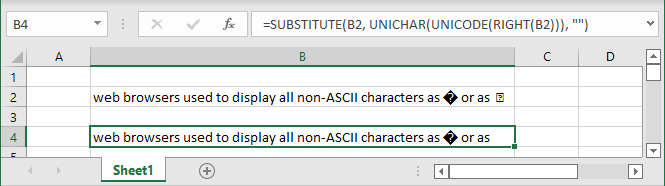# How to remove the diamond with question mark symbol

Excel
When you paste or import data into the Excel spreadsheet, you might notice unusual symbols like a diamond with a question mark. The replacement character � (often a black diamond with a white question mark or an empty square box) represents an unknown, unrecognized, or unrepresentable character: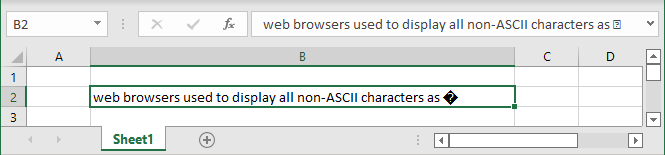Unfortunately, you can't remove these symbols using the standard Replace procedure. If you select this symbol and paste it into the Replace dialog box, you see the common space symbol: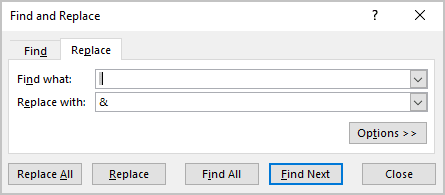Even more, if you try to replace it, you will see that Excel replaces all spaces in your spreadsheet: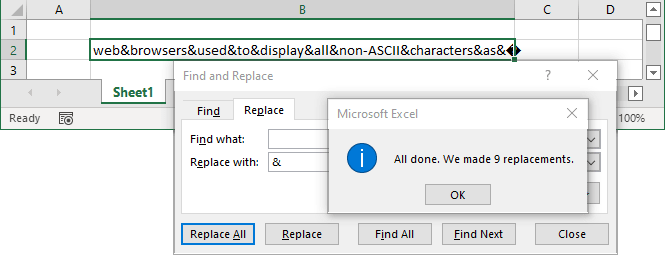To remove an unknown symbol from the cell or the entire spreadsheet, you need to find the code of this symbol and then substitute it.

## To remove the black diamond with the white question mark symbol

Try to use the formula:

= SUBSTITUTE (<cell>, UNICHAR (65533), “”):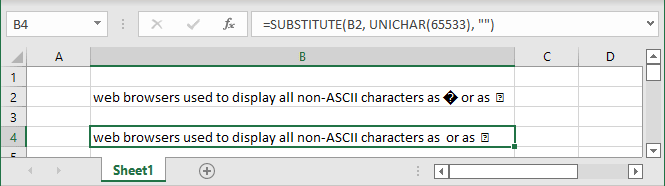where:

• The SUBSTITUTE (<text>, <old text>, <new text>) function substitutes <new text> for <old text> in a <text>.
• The UNICHAR (<numeric value>) function returns the Unicode character that is referenced by the given <numeric value>.

## To remove the rectangular with the question mark symbol

Try to use the formula:

= SUBSTITUTE (<cell>, UNICHAR (12), “”):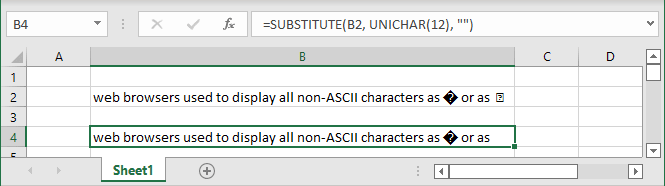## To remove any strange symbol from the cell

Use the formula:

= SUBSTITUTE (<cell>, UNICHAR (UNICODE (<character>)), “”):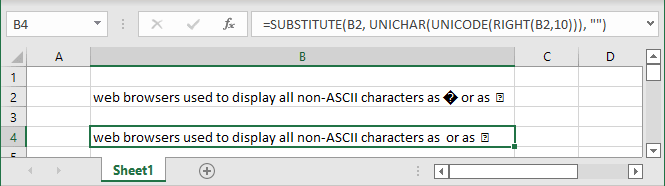where:

• The UNICODE (<character>) function returns the number (code point) corresponding to the first character of the text: